# Algebra Solving Linear Equations To solve linear equations

• Slides: 14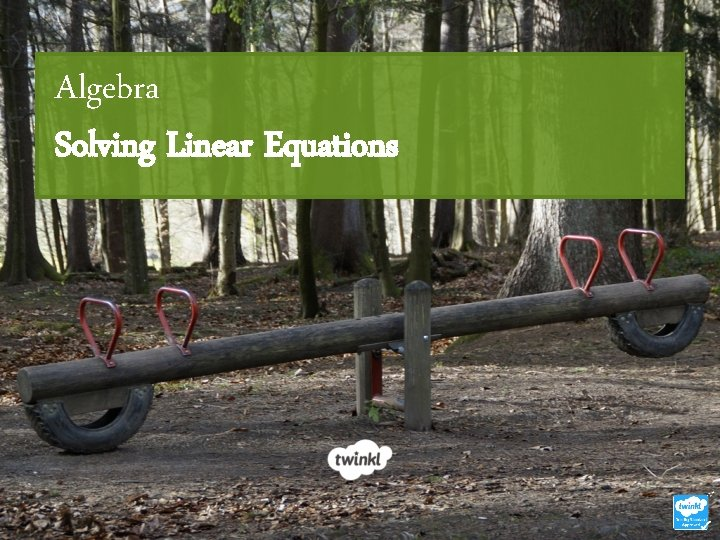Algebra Solving Linear EquationsTo solve linear equations. Learning Objective Success Criteria • To solve linear equations with an unknown on one side. • To solve linear equations involving brackets and fractions. • To create and then solve linear equations.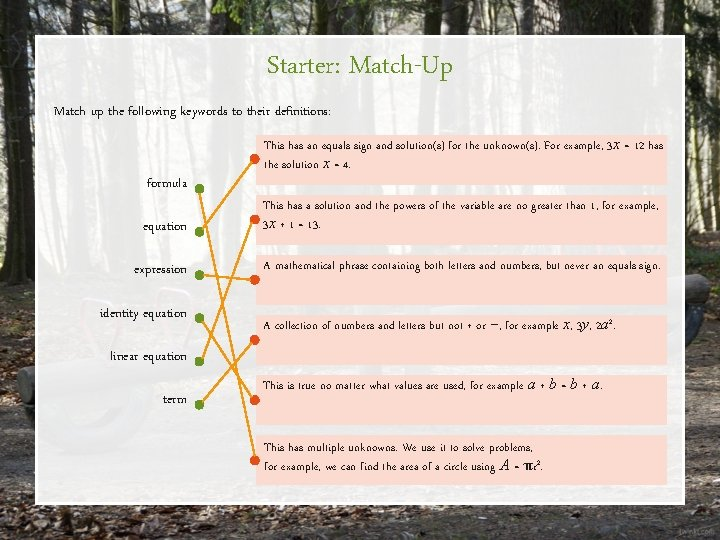Starter: Match-Up Match up the following keywords to their definitions: formula This has an equals sign and solution(s) for the unknown(s). For example, 3 x = 12 has the solution x = 4. equation This has a solution and the powers of the variable are no greater than 1, for example, 3 x + 1 = 13. expression A mathematical phrase containing both letters and numbers, but never an equals sign. identity equation A collection of numbers and letters but not + or −, for example x, 3 y, 2 a 2. linear equation term This is true no matter what values are used, for example a + b = b + a. This has multiple unknowns. We use it to solve problems, for example, we can find the area of a circle using A = πr 2.Solving Linear Equations 8 + 8 = 24 There are 8 counters in each bag. We can also use algebra to solve this problem… 3 x = 24 ÷ 3 x=8 Assuming there are the same number of counters in each bag, how many counters does each bag hold?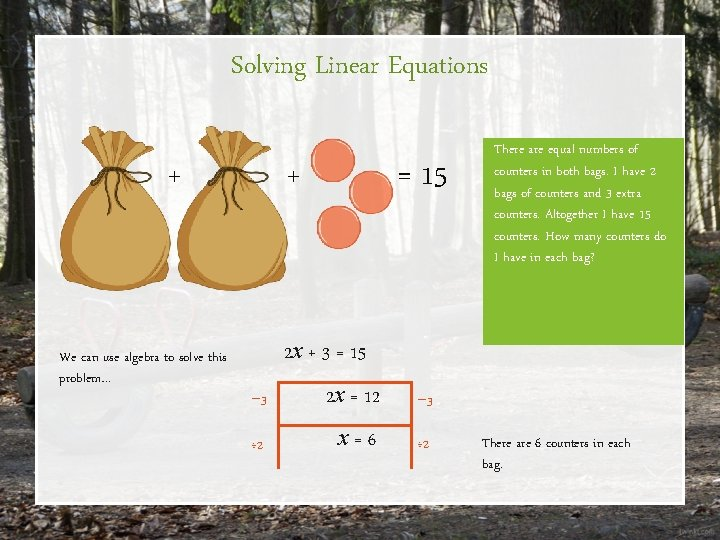Solving Linear Equations + We can use algebra to solve this problem… + = 15 There are equal numbers of counters in both bags. I have 2 bags of counters and 3 extra counters. Altogether I have 15 counters. How many counters do I have in each bag? 2 x + 3 = 15 − 3 2 x = 12 − 3 ÷ 2 x=6 ÷ 2 There are 6 counters in each bag.Solving Linear Equations with an Unknown on One Side Solve the following equation: a + 4 = 12 What is the question asking? It is asking us to find out the value of a. Something add 4 is equal to 12. Although this may seem straightforward and you can easily identify what the value of a is, we can also use something called ‘The Funnel Method’ to help us solve this equation. This method involves ‘funnelling’ out the known values and leaving the unknown on it’s own on one side of the equation. a +4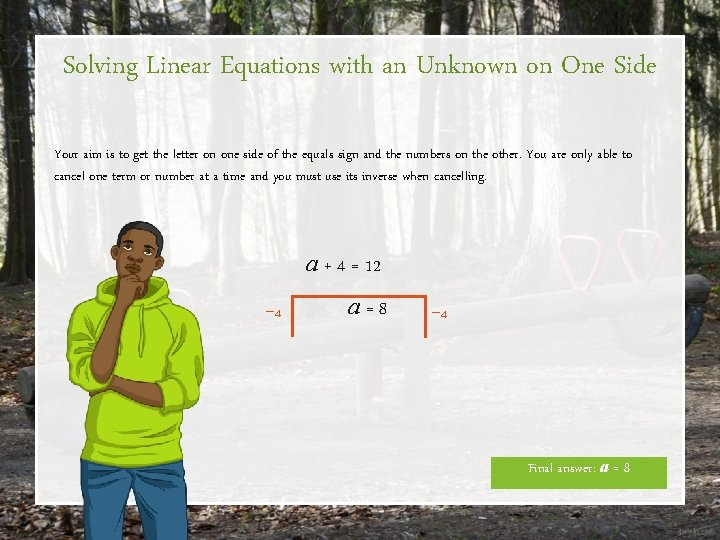Solving Linear Equations with an Unknown on One Side Your aim is to get the letter on one side of the equals sign and the numbers on the other. You are only able to cancel one term or number at a time and you must use its inverse when cancelling. a + 4 = 12 − 4 a=8 − 4 Final answer: a = 8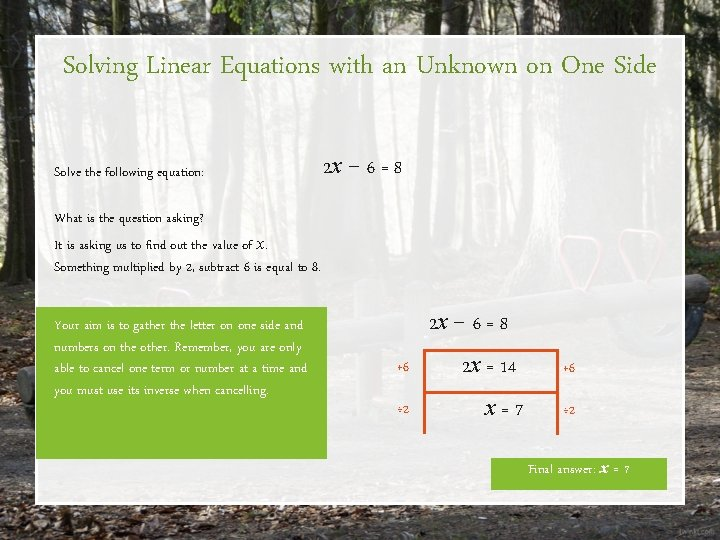Solving Linear Equations with an Unknown on One Side Solve the following equation: 2 x − 6 = 8 What is the question asking? It is asking us to find out the value of x. Something multiplied by 2, subtract 6 is equal to 8. Your aim is to gather the letter on one side and numbers on the other. Remember, you are only able to cancel one term or number at a time and you must use its inverse when cancelling. 2 x − 6 = 8 +6 ÷ 2 2 x = 14 x=7 +6 ÷ 2 Final answer: x = 7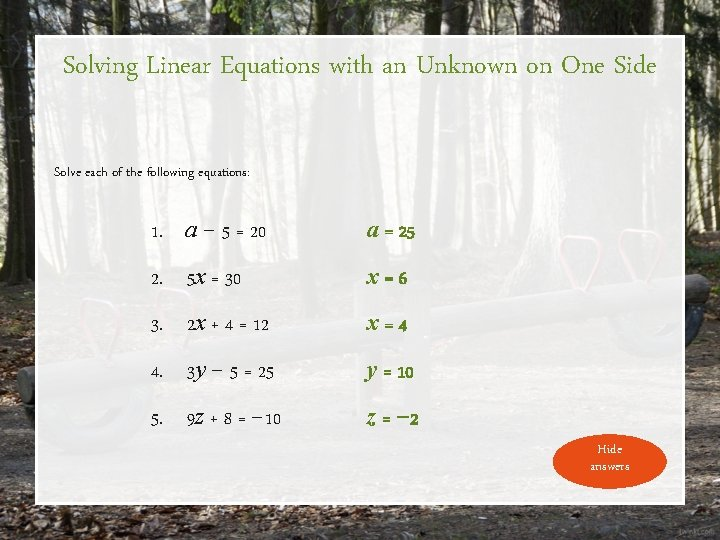Solving Linear Equations with an Unknown on One Side Solve each of the following equations: 1. a − 5 = 20 a = 25 2. 5 x = 30 x=6 3. 2 x + 4 = 12 x=4 4. 3 y − 5 = 25 y = 10 5. 9 z + 8 = − 10 z = − 2 Show Hide answers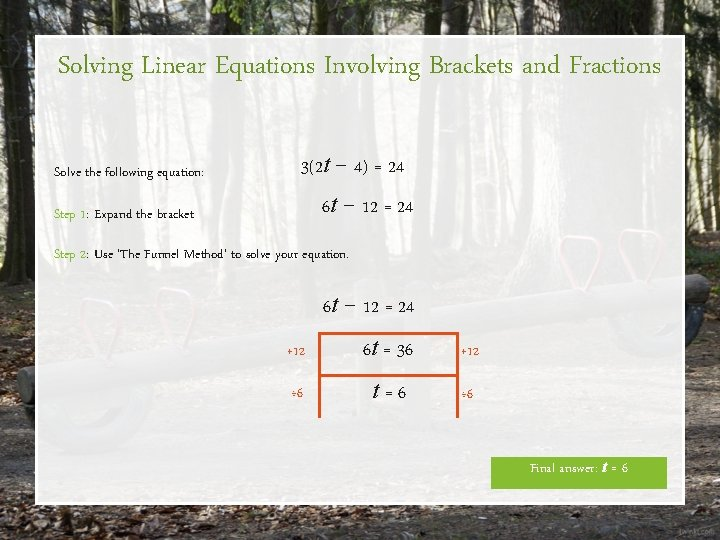Solving Linear Equations Involving Brackets and Fractions Solve the following equation: 3(2 t − 4) = 24 6 t − 12 = 24 Step 1: Expand the bracket Step 2: Use ‘The Funnel Method’ to solve your equation. 6 t − 12 = 24 +12 6 t = 36 +12 ÷ 6 t=6 ÷ 6 Final answer: t = 6Solving Linear Equations Involving Brackets and Fractions y + 1 = 12 3 Solve the following equation: Note that to cancel out the fraction, we must multiply by the denominator. − 1 × 3 y + 1 = 12 3 y = 11 3 y = 33 − 1 × 3 Final answer: y = 33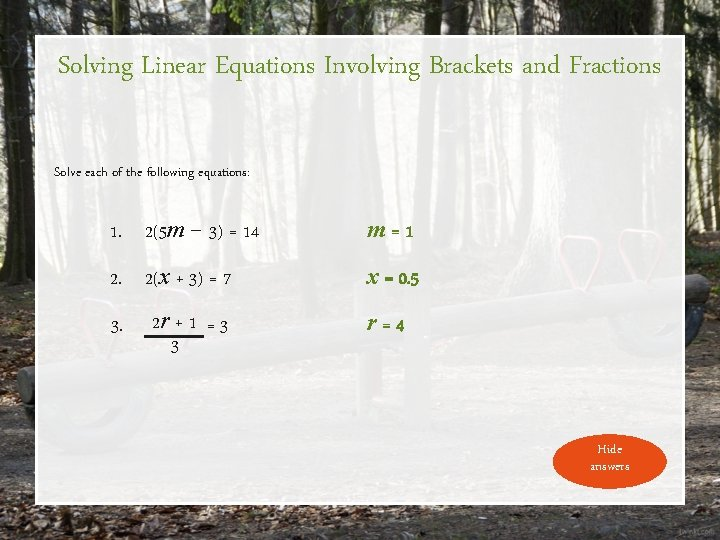Solving Linear Equations Involving Brackets and Fractions Solve each of the following equations: 1. 2(5 m − 3) = 14 m=1 2. 2(x + 3) = 7 x = 0. 5 3. 2 r + 1 = 3 3 r=4 Show Hide answers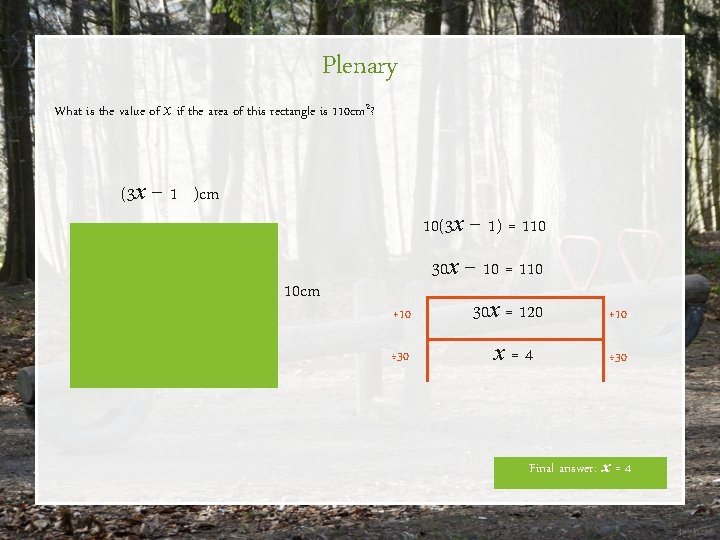Plenary What is the value of x if the area of this rectangle is 110 cm 2? (3 x − 1 )cm 10(3 x − 1) = 110 10 cm 30 x − 10 = 110 +10 ÷ 30 30 x = 120 x=4 +10 ÷ 30 Final answer: x = 4Photo courtesy of (@flickr. com) - granted under creative commons licence - attribution